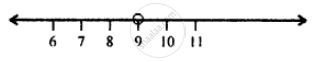# Solve and Graph the Solution Set on a Number Line: -3x + 12 < -15 ; X ∈ R. - Mathematics

Sum

Solve and graph the solution set on a number line: -3x + 12 < -15 ; x ∈ R.

#### Solution

-3x + 12 < -15

⇒ -3x + 12 - 12 < - 15 -12  (Subtracting 12 from both sides)

⇒ -3x < -27

⇒ (-"3x")/-3 > (-27)/(-3)  (Dividing both sides by -3)

Note: Division by a negative number reverses the inequality.

⇒ x > 9

∴ The required graph is:Concept: Operation on of Whole Number on Number Line
Is there an error in this question or solution?

#### APPEARS IN

Selina Concise Mathematics Class 8 ICSE
Chapter 15 Linear Inequations
Exercise 15 (B) | Q 3 | Page 175